Function Repository Resource:

# AllanDeviation

Measure the two-sample phase/frequency stability

Contributed by: Julien Kluge
 ResourceFunction["AllanDeviation"][data,r,taus] calculates the two-sample deviation of data along sample times taus at rate r.

## Details and Options

The Allan deviation is the square root of the two-sample variance, which is used to characterize the frequency/phase stability of an oscillator.
The output usually comes in the format {τ,Around[σ,δσ]} where δσ denotes the statistical uncertainty related to the deviation σ.
The argument taus can be specified as:
 {{n}} attempts to place n logarithmic spaced samples d places logarithmic spaced samplings with log base d {τ1,τ2,…} tries to use the specified sample times All uses all possible sample times Automatic tries to place a reasonable amount of samples along the whole possible time range
For a list of reals as a data argument, ResourceFunction["AllanDeviation"] will use a fast, compiled algorithm. Otherwise, valid inputs are exact expressions and/or Around.
The rate r must be a positive, numeric quantity.
Supported options are:
 "Overlapping" True whether the Allan deviation is overlapping "FrequencyData" False whether data is a list of frequencies, or False for phase data

## Examples

### Basic Examples (2)

Calculate the overlapping Allan deviation of white noise at a rate of 0.1 for automatically chosen sampling points:

 In:=Out=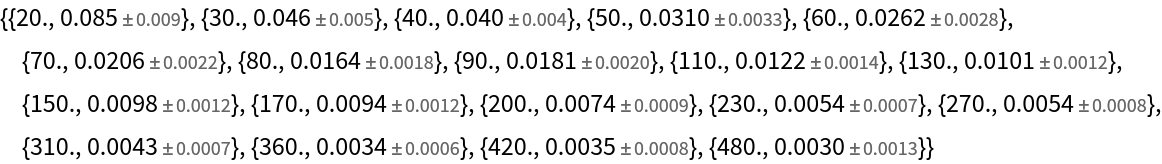Plot the results:

 In:=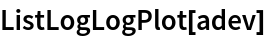Out=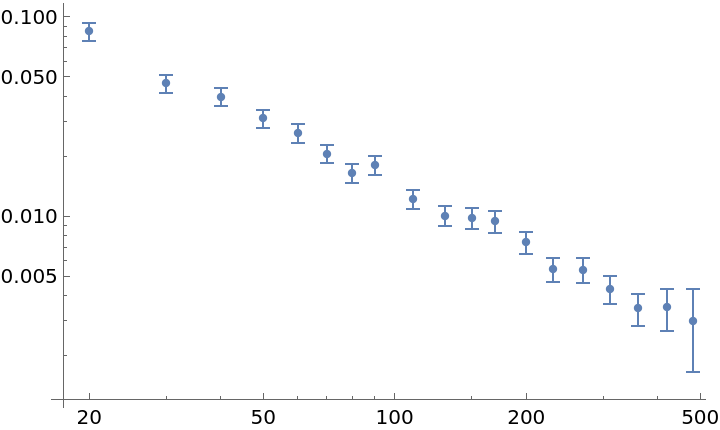Display the overlapping Allan deviation of white noise at a rate of 0.1 for specified sampling points:

 In:=Out=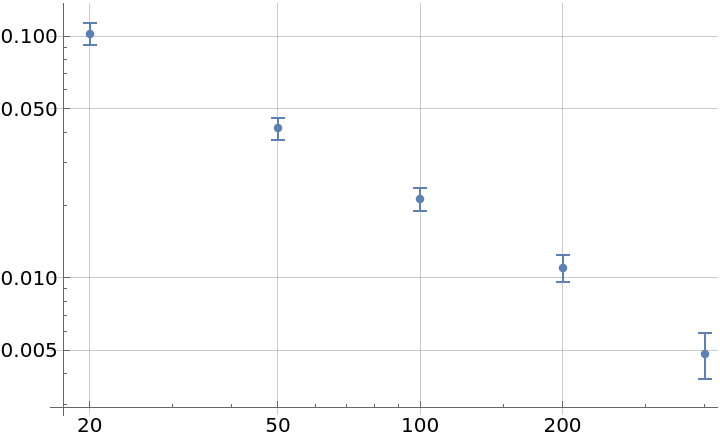### Scope (2)

Sample at all possible times:

 In:=Out=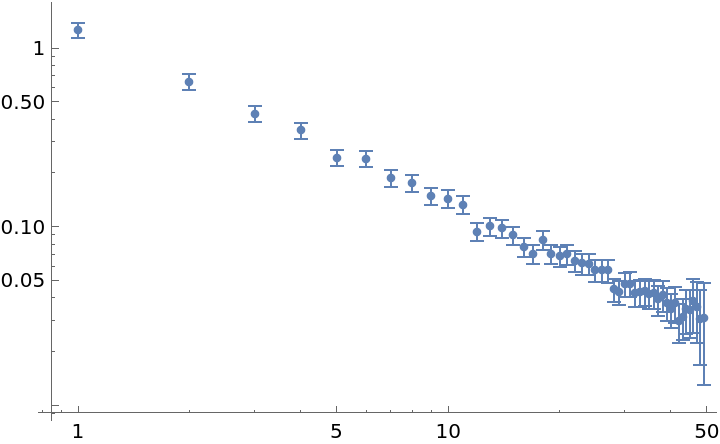Try to sample at five different time values:

 In:=Out=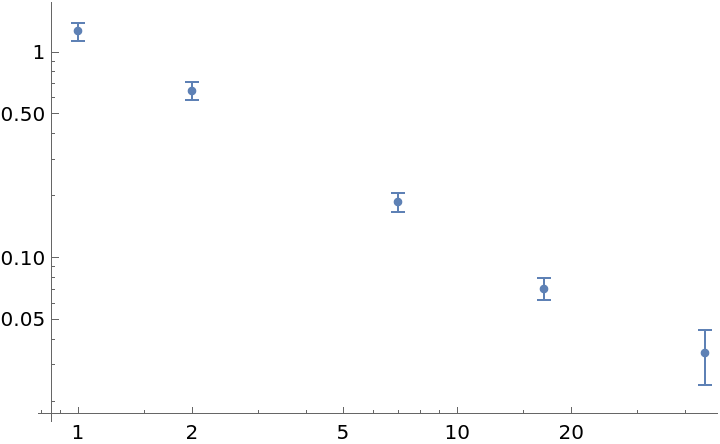The function will try to use a fast evaluation method when the data array is fully real, and otherwise prints a warning:

 In:=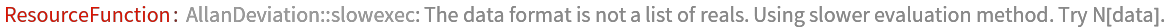Out=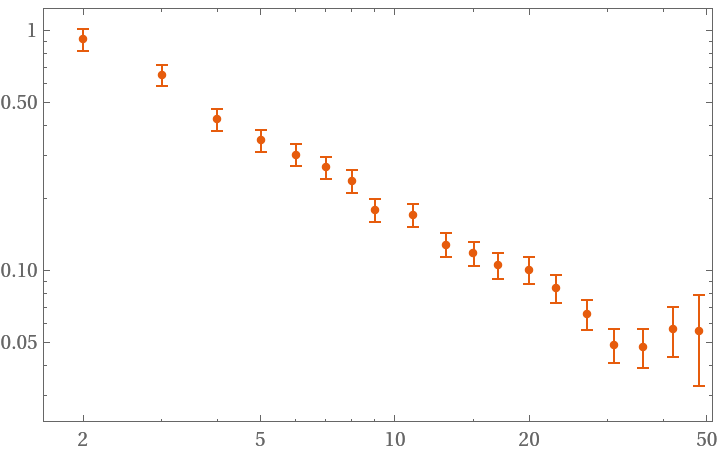### Options (2)

#### FrequencyData (1)

"FrequencyData" accepts a Boolean value. True signals the use of frequency data as opposed to phase data:

 In:=Out=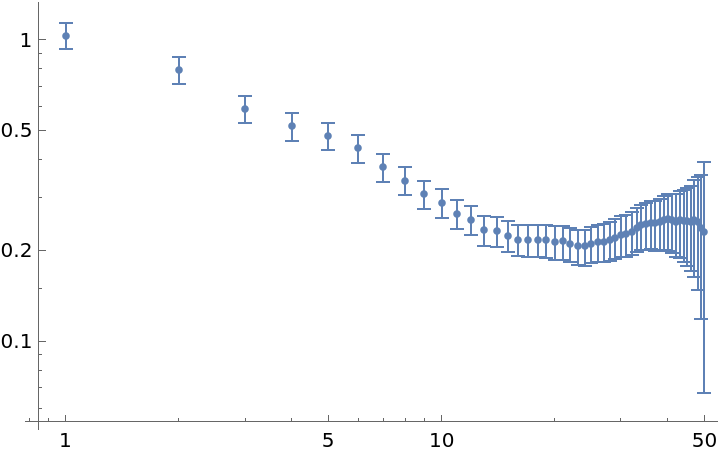#### Overlapping (1)

"Overlapping" accepts a Boolean value. False signals the use of non-overlapping strides in the deviation sampling:

 In:=Out=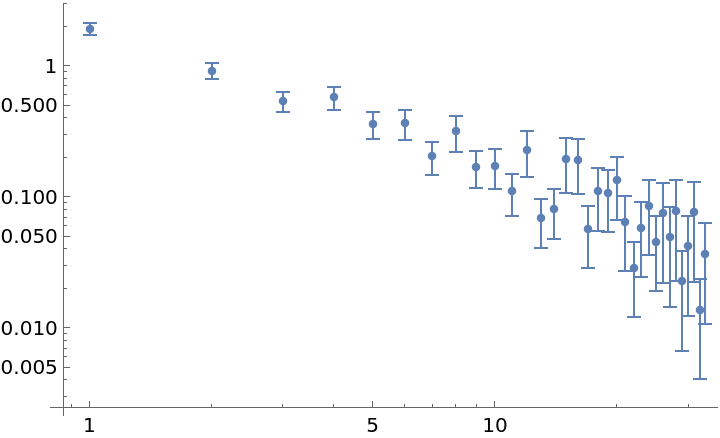### Applications (2)

Distinguish a random walk from white noise by their distinct slopes:

 In:=Out=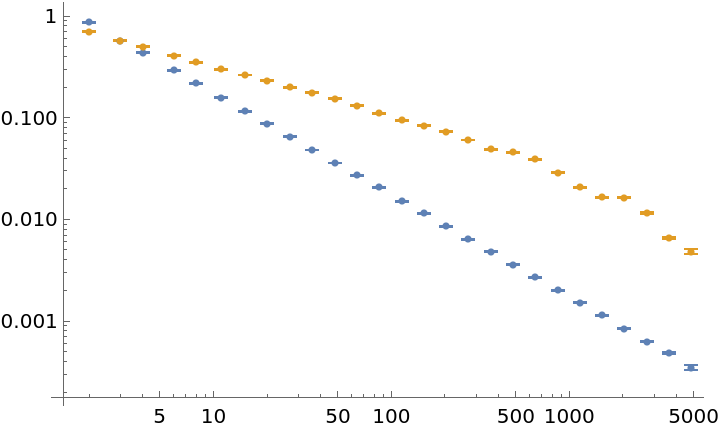Detect a drift in frequency data by a rising slope in longer sample times:

 In:=Out=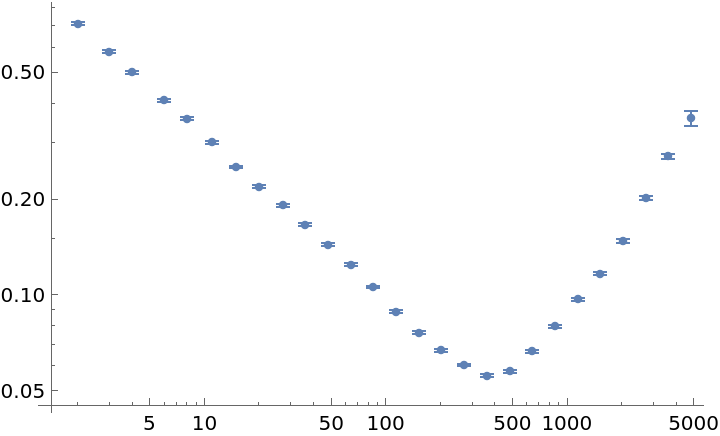### Properties and Relations (2)

Using the fast algorithm for numeric data is approximately two times faster:

 In:=Out=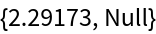In:=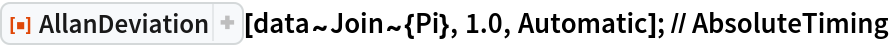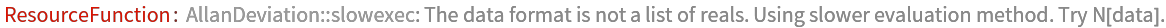Out=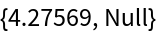The function can process Around objects as well as exact inputs directly:

 In:=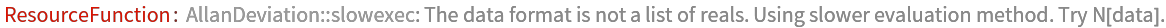Out=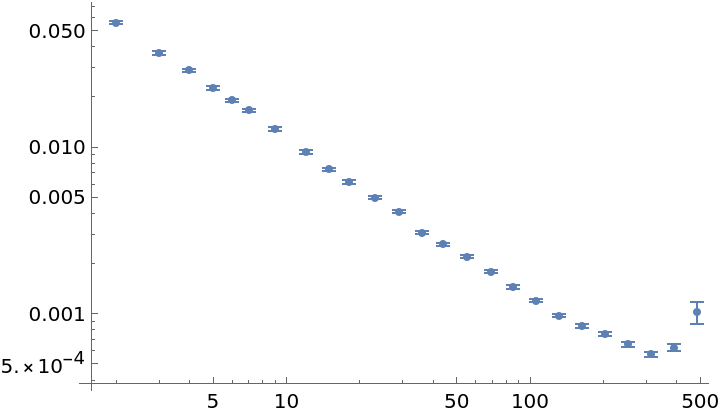### Possible Issues (1)

Requesting a certain number of samples will not necessarily result in this number of deviations:

 In:=Out=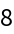Julien Kluge

## Version History

• 1.0.0 – 11 June 2020